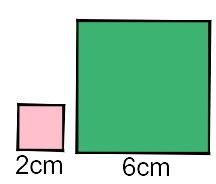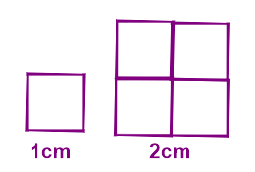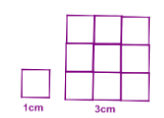# Calculate Sides and Areas of Similar Shapes Using Area Scale Factors

In this worksheet, students will identify similar shapes and use knowledge of proportions to work out the lengths of sides.Key stage:  KS 3

Curriculum topic:   Geometry and Measures

Curriculum subtopic:   Solve Problems Involving Perimeters and Areas of 2D Shapes

Difficulty level:#### Worksheet Overview

In this activity, we are going to be looking at the area scale factor of similar shapesWhat can we remember about scale factors?

A similar shape has the same size angles, but the sides are proportionally bigger or smaller.

We use scale factor to help us to work out any missing lengths.

For example, the squares below have sides of 2 cm and 6 cm.

Therefore, the sides are 6/2 = 3 times bigger on the green one.

This means that all the sides are 3 times bigger.

We call this the scale factor or sf.

So, the scale factor is 3.,

Area scale factor

This is best illustrated in a diagram:We can see that the squares above have corresponding sides 1 cm and 2 cm so the scale factor is 2.

The area is however 4 times bigger!

## In short, if we have the scale factor, we can square it to get the area scale factor!

Let's look at a question to help us understand it.

Example

Two similar shapes have a scale factor of 3.

What is the area scale factor?

As we said above, we can square the scale factor to get the area scale factor!Area scale factor = 32 = 9

You can see from the diagram above that the area of the bigger shape is 9 if the scale factor is 3.

Does that make sense?### What is EdPlace?

We're your National Curriculum aligned online education content provider helping each child succeed in English, maths and science from year 1 to GCSE. With an EdPlace account you’ll be able to track and measure progress, helping each child achieve their best. We build confidence and attainment by personalising each child’s learning at a level that suits them.

Get started